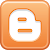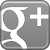# Sliding on Hover Jquery Featured Post Slider For Blogger

Every Blogger Dreams to make his blog attractive with perfect image content sliders. Usually image content sliders works on automation. So they don’t really allow you to select the certain post that you want to view. They keep on sliding the same thing in front of your eyes and in that situation you are unable to do any thing, which is biggest problem that you face with these content sliders. This was the reason why, in our previous post we introduced a Shuttingup and down Featured post Widget for Blogger.

But, today we will introduce a greatly new and improved Slide on Hover Feature Post Widget for Blogger with JQuery effect. It will change the entire reflection of your blog. In this widget you can add up to 5 featured posts with their large images, titles and little descriptions. So today we will going to Add Sliding on Hover JQuery Featured Post Widget for Blogger

### How This Slide on Hover Slider Widget Works:

This is not an ordinary slider, it is totally different from all other sliders which makes it unique. Basically this Slide on Hover Slider consist of 5 featured posts and when ever you'll place your mouse on any certain post in the slider, it will expand the post that you have selected.

So I am certain you would love to see the live demo, so here it is give it a try before adding it to your blog.
I am sure after viewing the live demo you would love to add it in your blog. So without wasting any time let us start our mouse rolling on our tutorial.

### Adding Style Sheet CSS to Blogger Template:

In every widget CSS plays anchor role, so first we will insert style sheet to our blogger template
• So Go To Blogger >> Your Blog
• Now Click Template
• Now Backup Your template (Incase any thing goes wrong)
• Now Click on Proceed >> Edit HTML
• Now Search for the Following Code in your template with (CTRL+F Shortcut to Search)
]]></b:skin>
• So when you found ]]></b:skin> then just above it paste the following code.

/* MBL-Slide-On-Hover-Featured-Slider-Widget */
#slider {
border:1px solid #aaa;
border-top:none;
margin: 0;
width: 957px;
height: 320px;
overflow: hidden;
.kwicks { position:relative }
.kwicks li{
width: 192px;
height: 320px;
display: block;
overflow: hidden;
border-right:1px solid #aaa;
display: block;
position: absolute;
right: 0;
width: 300px;
height: 320px;
z-index: 4;
background: url(https://lh6.googleusercontent.com/-LNAjyaTqLpk/Tf_MHeJND-I/AAAAAAAAA8M/JvjGU6SPB2Q/overlay.png) repeat-y scroll top right transparent; }
.kwicks.horizontal li { margin-right: 0px; float: left; }
.kwicks.vertical  li { margin-bottom: 5px; }
.kwicks .excerpt {
background: #fff;
color: #000;
opacity: 0.9;
filter:alpha(opacity=90);
position: absolute;
bottom: 0px;
display: block;
width: 100%; }
.excerpt strong {
font-family: "Trebuchet MS",Arial,Helvetica,san-serif;
font-weight: normal;
letter-spacing: 0.4px;
height: 76px;
line-height: 26px;
font-size: 14px;
display:block;
text-transform:uppercase; }
• Now After Pasting the above code in your template save your template by pressing Save Template button

### Adding JQuery Scripts To Blogger Template:

Now we will add Jquery Scripts in our template to do so.
• Go to Blogger >> Your Blog >>
• Now Select >> Add HTML/JavaScript >> And then just paste the following code there

<script type='text/javascript'>//<![CDATA[
jQuery.easing['jswing']=jQuery.easing['swing'];jQuery.extend(jQuery.easing,{def:'easeOutQuad',swing:function(x,t,b,c,d){return jQuery.easing[jQuery.easing.def](x,t,b,c,d);},easeInQuad:function(x,t,b,c,d){return c*(t/=d)*t+b;},easeOutQuad:function(x,t,b,c,d){return-c*(t/=d)*(t-2)+b;},easeInOutQuad:function(x,t,b,c,d){if((t/=d/2)<1)return c/2*t*t+b;return-c/2*((--t)*(t-2)-1)+b;},easeInCubic:function(x,t,b,c,d){return c*(t/=d)*t*t+b;},easeOutCubic:function(x,t,b,c,d){return c*((t=t/d-1)*t*t+1)+b;},easeInOutCubic:function(x,t,b,c,d){if((t/=d/2)<1)return c/2*t*t*t+b;return c/2*((t-=2)*t*t+2)+b;},easeInQuart:function(x,t,b,c,d){return c*(t/=d)*t*t*t+b;},easeOutQuart:function(x,t,b,c,d){return-c*((t=t/d-1)*t*t*t-1)+b;},easeInOutQuart:function(x,t,b,c,d){if((t/=d/2)<1)return c/2*t*t*t*t+b;return-c/2*((t-=2)*t*t*t-2)+b;},easeInQuint:function(x,t,b,c,d){return c*(t/=d)*t*t*t*t+b;},easeOutQuint:function(x,t,b,c,d){return c*((t=t/d-1)*t*t*t*t+1)+b;},easeInOutQuint:function(x,t,b,c,d){if((t/=d/2)<1)return c/2*t*t*t*t*t+b;return c/2*((t-=2)*t*t*t*t+2)+b;},easeInSine:function(x,t,b,c,d){return-c*Math.cos(t/d*(Math.PI/2))+c+b;},easeOutSine:function(x,t,b,c,d){return c*Math.sin(t/d*(Math.PI/2))+b;},easeInOutSine:function(x,t,b,c,d){return-c/2*(Math.cos(Math.PI*t/d)-1)+b;},easeInExpo:function(x,t,b,c,d){return(t==0)?b:c*Math.pow(2,10*(t/d-1))+b;},easeOutExpo:function(x,t,b,c,d){return(t==d)?b+c:c*(-Math.pow(2,-10*t/d)+1)+b;},easeInOutExpo:function(x,t,b,c,d){if(t==0)return b;if(t==d)return b+c;if((t/=d/2)<1)return c/2*Math.pow(2,10*(t-1))+b;return c/2*(-Math.pow(2,-10*--t)+2)+b;},easeInCirc:function(x,t,b,c,d){return-c*(Math.sqrt(1-(t/=d)*t)-1)+b;},easeOutCirc:function(x,t,b,c,d){return c*Math.sqrt(1-(t=t/d-1)*t)+b;},easeInOutCirc:function(x,t,b,c,d){if((t/=d/2)<1)return-c/2*(Math.sqrt(1-t*t)-1)+b;return c/2*(Math.sqrt(1-(t-=2)*t)+1)+b;},easeInElastic:function(x,t,b,c,d){var s=1.70158;var p=0;var a=c;if(t==0)return b;if((t/=d)==1)return b+c;if(!p)p=d*.3;if(a<Math.abs(c)){a=c;var s=p/4;}
else var s=p/(2*Math.PI)*Math.asin(c/a);return-(a*Math.pow(2,10*(t-=1))*Math.sin((t*d-s)*(2*Math.PI)/p))+b;},easeOutElastic:function(x,t,b,c,d){var s=1.70158;var p=0;var a=c;if(t==0)return b;if((t/=d)==1)return b+c;if(!p)p=d*.3;if(a<Math.abs(c)){a=c;var s=p/4;}
else var s=p/(2*Math.PI)*Math.asin(c/a);return a*Math.pow(2,-10*t)*Math.sin((t*d-s)*(2*Math.PI)/p)+c+b;},easeInOutElastic:function(x,t,b,c,d){var s=1.70158;var p=0;var a=c;if(t==0)return b;if((t/=d/2)==2)return b+c;if(!p)p=d*(.3*1.5);if(a<Math.abs(c)){a=c;var s=p/4;}
else var s=p/(2*Math.PI)*Math.asin(c/a);if(t<1)return-.5*(a*Math.pow(2,10*(t-=1))*Math.sin((t*d-s)*(2*Math.PI)/p))+b;return a*Math.pow(2,-10*(t-=1))*Math.sin((t*d-s)*(2*Math.PI)/p)*.5+c+b;},easeInBack:function(x,t,b,c,d,s){if(s==undefined)s=1.70158;return c*(t/=d)*t*((s+1)*t-s)+b;},easeOutBack:function(x,t,b,c,d,s){if(s==undefined)s=1.70158;return c*((t=t/d-1)*t*((s+1)*t+s)+1)+b;},easeInOutBack:function(x,t,b,c,d,s){if(s==undefined)s=1.70158;if((t/=d/2)<1)return c/2*(t*t*(((s*=(1.525))+1)*t-s))+b;return c/2*((t-=2)*t*(((s*=(1.525))+1)*t+s)+2)+b;},easeInBounce:function(x,t,b,c,d){return c-jQuery.easing.easeOutBounce(x,d-t,0,c,d)+b;},easeOutBounce:function(x,t,b,c,d){if((t/=d)<(1/2.75)){return c*(7.5625*t*t)+b;}else if(t<(2/2.75)){return c*(7.5625*(t-=(1.5/2.75))*t+.75)+b;}else if(t<(2.5/2.75)){return c*(7.5625*(t-=(2.25/2.75))*t+.9375)+b;}else{return c*(7.5625*(t-=(2.625/2.75))*t+.984375)+b;}},easeInOutBounce:function(x,t,b,c,d){if(t<d/2)return jQuery.easing.easeInBounce(x,t*2,0,c,d)*.5+b;return jQuery.easing.easeOutBounce(x,t*2-d,0,c,d)*.5+c*.5+b;}});

/*
Kwicks for jQuery (version 1.5.1)
http://www.jeremymartin.name/projects.php?project=kwicks
Any and all use of this script must be accompanied by this copyright/license notice in its present form.
*/
(function(\$){\$.fn.kwicks=function(n){var p={isVertical:false,sticky:false,defaultKwick:0,event:'mouseover',spacing:0,duration:500};var o=\$.extend(p,n);var q=(o.isVertical?'height':'width');var r=(o.isVertical?'top':'left');return this.each(function(){container=\$(this);var k=container.children('li');var l=k.eq(0).css(q).replace(/px/,'');if(!o.max){o.max=(l*k.size())-(o.min*(k.size()-1))}else{o.min=((l*k.size())-o.max)/(k.size()-1)}if(o.isVertical){container.css({width:k.eq(0).css('width'),height:(l*k.size())+(o.spacing*(k.size()-1))+'px'})}else{container.css({width:(l*k.size())+(o.spacing*(k.size()-1))+'px',height:k.eq(0).css('height')})}var m=[];for(i=0;i<k.size();i++){m[i]=[];for(j=1;j<k.size()-1;j++){if(i==j){m[i][j]=o.isVertical?j*o.min+(j*o.spacing):j*o.min+(j*o.spacing)}else{m[i][j]=(j<=i?(j*o.min):(j-1)*o.min+o.max)+(j*o.spacing)}}}k.each(function(i){var h=\$(this);if(i===0){h.css(r,'0px')}else if(i==k.size()-1){h.css(o.isVertical?'bottom':'right','0px')}else{if(o.sticky){h.css(r,m[o.defaultKwick][i])}else{h.css(r,(i*l)+(i*o.spacing))}}if(o.sticky){if(o.defaultKwick==i){h.css(q,o.max+'px');h.addClass('active')}else{h.css(q,o.min+'px')}  }h.css({margin:0,position:'absolute'});h.bind(o.event,function(){var c=[];var d=[];k.stop().removeClass('active');for(j=0;j<k.size();j++){c[j]=k.eq(j).css(q).replace(/px/,'');d[j]=k.eq(j).css(r).replace(/px/,'')}var e={};e[q]=o.max;var f=o.max-c[i];var g=c[i]/f;h.addClass('active').animate(e,{step:function(a){var b=f!=0?a/f-g:1;k.each(function(j){if(j!=i){k.eq(j).css(q,c[j]-((c[j]-o.min)*b)+'px')}if(j>0&&j<k.size()-1){k.eq(j).css(r,d[j]-((d[j]-m[i][j])*b)+'px')}})},duration:o.duration,easing:o.easing})})});if(!o.sticky){container.bind("mouseleave",function(){var c=[];var d=[];k.removeClass('active').stop();for(i=0;i<k.size();i++){c[i]=k.eq(i).css(q).replace(/px/,'');d[i]=k.eq(i).css(r).replace(/px/,'')}var e={};e[q]=l;var f=l-c;k.eq(0).animate(e,{step:function(a){var b=f!=0?(a-c)/f:1;for(i=1;i<k.size();i++){k.eq(i).css(q,c[i]-((c[i]-l)*b)+'px');if(i<k.size()-1){k.eq(i).css(r,d[i]-((d[i]-((i*l)+(i*o.spacing)))*b)+'px')}}},duration:o.duration,easing:o.easing})})}})}})(jQuery);
\$('.kwicks').kwicks({
max : 700,
easing: 'easeOutQuart',
spacing : 0,
});
});

//]]></script>
• Now After pasting the above code save the gadget by pressing Save button.

### Inserting The Basic Html Of slider:

Now we will insert the most important thing, which is the basic HTML. It is the complete structure of our widget. Where we will insert all of our featured post details form images to description.
• So Go To Blogger >> Your Blog >>
• Now Select >> Add HtML/Javascript >> And now just paste the following code there
<ul class="kwicks horizontal" >
<li id="kwick_1">
<div class="excerpt">
<strong>Your-First-Post-Title-Here-With-Description</strong></div>
<a href="Your-First-Post-Url-Here" rel="bookmark"><img src="Your-First-Image-Url-Here" width="700" height="320" /></a></li>

<li id="kwick_2">
<div class="excerpt">
<strong>Your-Second-Post-Title-Here-With-Description</strong></div>
<a href="Your-Second-Post-Url-Here" rel="bookmark"><img src="Your-Second-Image-Url-Here" width="700" height="320" /></a>
</li>

<li id="kwick_3">
<div class="excerpt">
<strong>Your-Third-Post-Title-Here-With-Description</strong></div>
<a href="Your-Third-Post-Url-Here" rel="bookmark"><img src="Your-Third-Image-Url-Here" width="700" height="320" /></a></li>

<li id="kwick_4">
<div class="excerpt">
<strong>Your-Fourth-Post-Title-Here-With-Description</strong></div>
<a href="Your-Fourth-Post-Url-Here" rel="bookmark"><img src="Your-Fourth-Image-Url-Here" width="700" height="320" /></a></li>

<li id="kwick_5">
<div class="excerpt">
<strong>Your-Fifth-Post-Title-Here-With-Description</strong></div>
<a href="Your-Fifth-Post-Url-Here" rel="bookmark"><img src="Your-Fifth-Image-Url-Here" width="700" height="320" /></a></li></ul>

### Customization:

This widget contain 5 featured post, so you need to configure it for title of the post with the description, The image of your post and the Url of your post.

To Customize your First Featured Post:
Replace Your-First-Post-Title-Here-With-Description with your post title and description.
Replace Your-First-Image-Url-Here with the URL of your image
Replace Your-First-Post-Url-Here with your post URL

To Customize your Second Featured Post:
Replace Your-Second-Post-Title-Here-With-Description with your post title & description.
Replace Your-Second-Image-Url-Here with the URL of your image
Replace Your-Second-Post-Url-Here with your post URL

To Customize your Third Featured Post:
Replace Your-Third-Post-Title-Here-With-Description with your post title and description.
Replace Your-Third-Image-Url-Here with the URL of your image
Replace Your-Third-Post-Url-Here with your post URL

To Customize your Fourth Featured Post:
Replace Your-Fourth-Post-Title-Here-With-Description with your post title and description.
Replace Your-Fourth-Image-Url-Here with the URL of your image
Replace Your-Fourth-Post-Url-Here with your post URL

To Customize your Fifth Featured Post:
Replace Your-Fifth-Post-Title-Here-With-Description with your post title and description.
Replace Your-Fifth-Image-Url-Here with the URL of your image
Replace Your-Fifth-Post-Url-Here with your post URL

• Now After Customizing all five Featured Posts,Press the Save Button and View the Blog it would be perfect photo finish!!!!!
Note:Your image that you will going to link in the featured post should have  Width 700px and the height  320px to gave most desirable results.

The slider is still very much new, if you feel any difficulties in adding this widget to your blog feel free to ask. I would love to help you. So thats it for now, till then peace blessings and happy sliding :)The Editorial Team of MyBloggerLab consists of a group of Professional Blogger geeks Led by Syed Faizan Ali (Founder of MyBloggerLab).

##### Comment With:##### OR##### Choose Wisely!

April 22, 2012 at 7:39 PM

it not recommend to add this type of jquery widget..these widget can affect your blog's speed.. m i right?

Editorial Team MOD
April 22, 2012 at 8:30 PM

@Prince@Hunk

Well It does not Effect The Speed Of a website. And MyBloggerLab is an example of that. You Can See we are using the Same Scripts, and still our site speed is relativity Faster. So I in my opinion neglecting this widget for speed issues does not make sense. So I Recommend Every One to use it. Peace And Blessing Pal

Editorial Team MOD
April 23, 2012 at 1:43 AM

@Prince@Hunk

No Problem Pal, This blog Is Yours :). So Never Shy To Ask.Peace and Blessing buddyAnonymous
April 25, 2012 at 2:23 PM

its not working in my blog. why need to add two html gadgets? my blog sample is: http://a1b2c3-dayana.blogspot.in/

Editorial Team MOD
April 25, 2012 at 8:23 PM

@Dayana

Thanks, Please Follow the steps correctly and it will work perfect. And The First Html Gadget we added was JavaScript And Secound HTML Gadget is Basic Html Structure Which Gives Life To Our Gadget Which Consists Of Links And Images. I checked your blog but i was unable to see this gadget. Try To Apply it Correctly..Peace And Blessings

May 1, 2012 at 3:04 AM

Is There Any Widget Does Automatically Updates New Posts As Featured Posts....It Should Be Slider.....Thanks In Advance

Editorial Team MOD
May 1, 2012 at 9:38 AM

@Ramakrishna Ch
Brother there are some widgets which operate automatically with the help of RSS Feeds. But there are no Image Slider widgets which have this feature. But there are some Photo Gallery widgets Which updates automatically. But they don't have a Sliding effect instead they are optimized with Glow and vanish effect. Peace and Blessing Buddy

May 1, 2012 at 9:41 AM

can you provide me the photo gallery widgets which updates automatically

Editorial Team MOD
May 1, 2012 at 9:47 AM

@Ramakrishna Ch
Yeah Brother we will, Instead we will share a Complete Step by Step Tutorial For your convenience as MyBloggerLab is for readers like you :). Peace Pal

May 1, 2012 at 9:48 AM

thank you for your instant help

Editorial Team MOD
May 1, 2012 at 9:50 AM

@Ramakrishna Ch
No problem Pal, Its Our Pleasure. If you have any Problem at any time feel free to Ask. Peace

May 7, 2012 at 5:56 PM

thanks to this post. its not working to my testing blog but it only show the images though theres no animation. Here you can view this. I inserted above.

testing blog
http://testplugins.blogspot.com/

Editorial Team MOD
May 8, 2012 at 12:24 AM

@Mark AlonzoAnonymous
May 30, 2012 at 12:34 AM

Nice tip, thanks :)

June 7, 2012 at 8:03 PM

http://tulongankita.blogspot.com/

this my blog ... the way to put proper, help me blogmaster. :D

July 22, 2012 at 5:03 AM

Hey! There :) How are you? well I tried to make it work on MBT clone but its not I followed all of the above 3 steps but still not working you got the same template customized mb you can help! :) peace.
The blog em tryng to make it work on: BLOG

My Blog, Widgets,Templates,Giveaways, Reviews and more.
Blogger Freaks

Editorial Team MOD
July 22, 2012 at 6:01 AM

Hey Buddy! Yes we were using MBT PRO Template (Not the clone One) So Its pretty obvious that we can easily integrate this widget on that template. Please Send the Template to Contact.MyBloggerLab.com and we will take care of it. Peace and Blessings!!!

July 22, 2012 at 6:16 AM

Hmm! I have emailed you the template buddy!

Editorial Team MOD
July 22, 2012 at 6:20 AM

OK Dude! Wait Until we put things together.

July 23, 2012 at 8:52 AM

dude i think there is a problem with your coding that's why, like you can see most of the people are having problem implementing it can you please check the codes.

Editorial Team MOD
July 23, 2012 at 10:28 AM

Yes Mate! We agree its not working for few custom blogger template our best man are working to get everything perfect. We will update the Coding, till then stay tuned.

July 25, 2012 at 1:01 PM

its working, but why the discription only appeared in the first featured post only..???

http://ibnualjufri24.blogspot.com/

Editorial Team MOD
July 25, 2012 at 1:04 PM

Hey Bro! I Checked Your Blog that's might be happening because there are tons of widgets on your blog so two scripts are conflicting with each other which is causing some Bugs. However, We are updating the Slider to make it more easier and Better. Peace and Blessings

July 25, 2012 at 2:04 PM

Ok bro...thankz...Anonymous
September 23, 2012 at 4:17 AM

how i can adjust the width

December 25, 2012 at 1:42 AM

Its not working :(

April 27, 2013 at 1:09 AM

Hey Nice Wodget. Now can you tell me how can I add Links to the title so that users can click and read more?. Reply Fast

Editorial Team MOD
April 27, 2013 at 1:46 AM

Every thing is mentioned in the tutorial check it properly.

July 11, 2013 at 1:39 AM

Is it possible to add more than five posts, i added but its goes out of the page, any solutions?